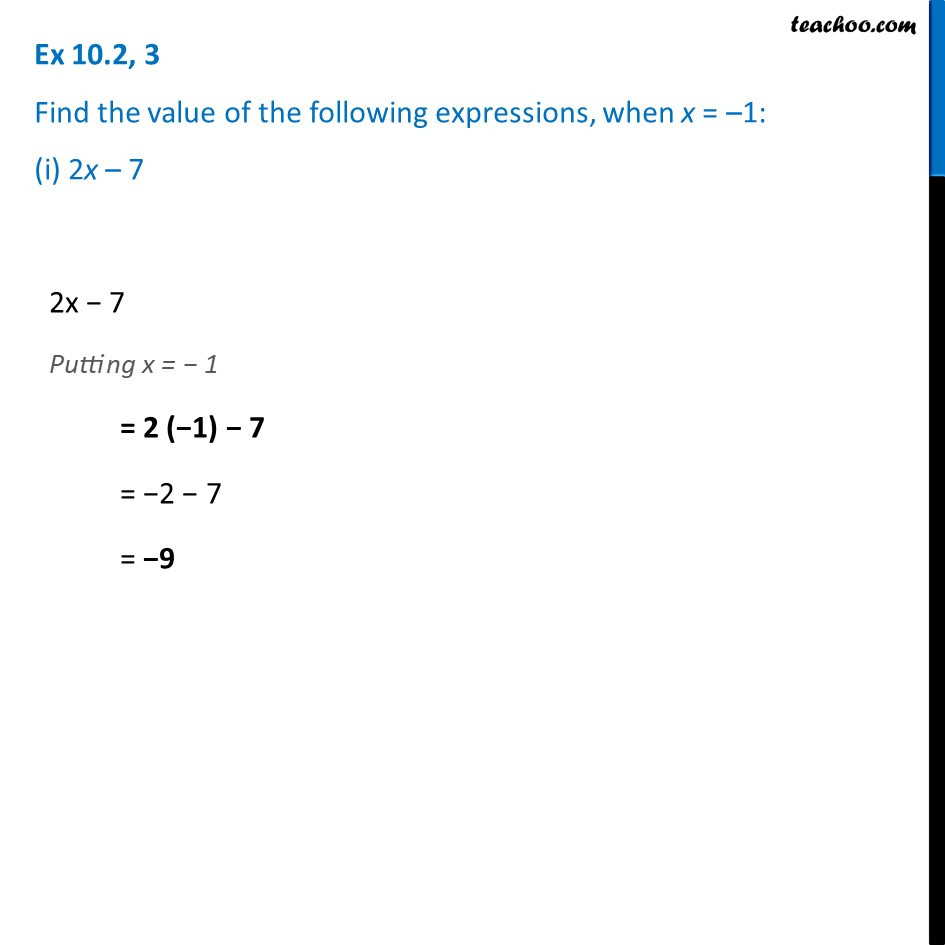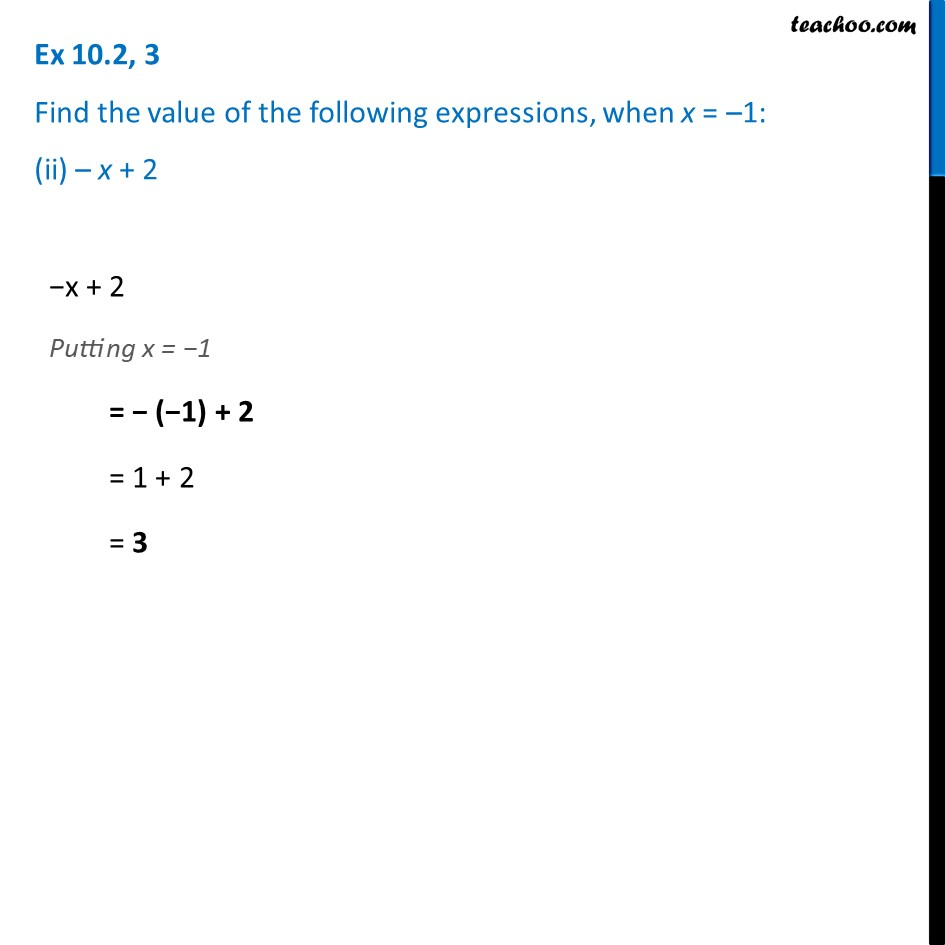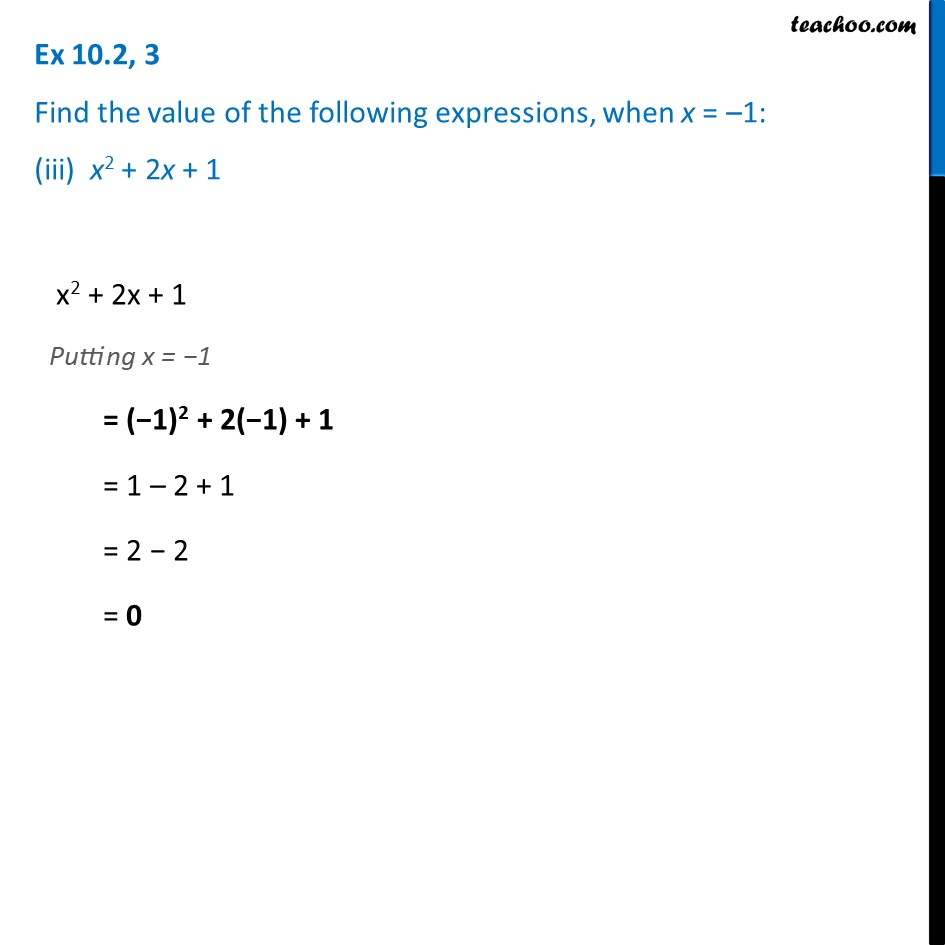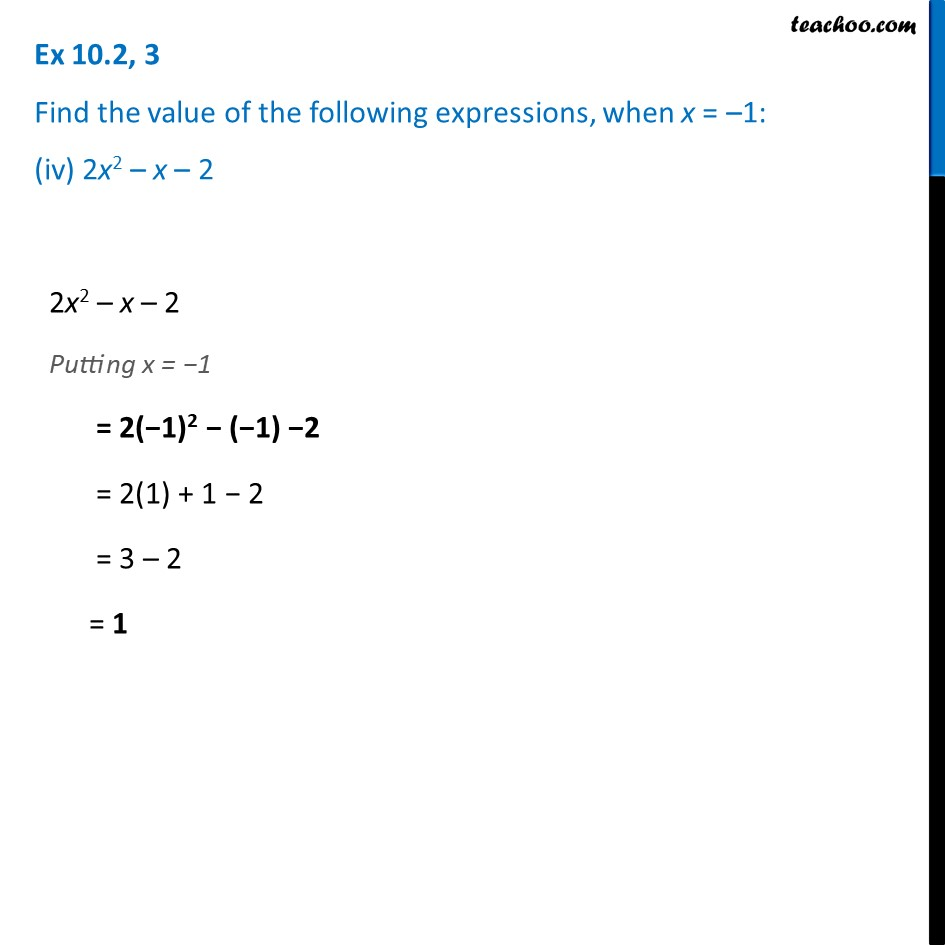Ex 10.2

Chapter 10 Class 7 Algebraic Expressions
Serial order wiseLearn in your speed, with individual attention - Teachoo Maths 1-on-1 Class

### Transcript

Ex 10.2, 3 Find the value of the following expressions, when x = –1: (i) 2x – 72x − 7 Putting x = − 1 = 2 (−1) − 7 = −2 − 7 = −9 −x + 2 Putting x = −1 = − (−1) + 2 = 1 + 2 = 3 Ex 10.2, 3 Find the value of the following expressions, when x = –1: (ii) – x + 2Ex 10.2, 3 Find the value of the following expressions, when x = –1: (iii) x2 + 2x + 1 x2 + 2x + 1 Putting x = −1 = (−1)2 + 2(−1) + 1 = 1 – 2 + 1 = 2 − 2 = 0 Ex 10.2, 3 Find the value of the following expressions, when x = –1: (iv) 2x2 – x – 22x2 – x – 2 Putting x = −1 = 2(−1)2 − (−1) −2 = 2(1) + 1 − 2 = 3 – 2 = 1